Vignette 15
Julia Sets

Fractals are sets that are self-similar and have detail on all levels of magnification.  Vignette 3 introduced the snowflake curve and other fractal sets that can be constructed using a geometric technique called iterated subset replacement.  In this Vignette, we will look at a very different way of constructing certain fractal sets known as Julia sets.

Prisoners and Escapees

The pioneering work of the French mathematician Gaston Julia, published in 1918 when Julia was 25 years old, was essentially forgotten by the mathematical world until the 1980's, when computers made possible the visualization of his creation.  Julia's idea was to observe the behavior of the orbit of a complex number (see Vignette 6 and Vignette 10) under iteration of a function f .  That is, begin with a complex number z0 , visualized as a point in the plane, and apply to z0 .  The resulting value is fed back into the function f  to obtain a new complex number z1.  This in turn is fed back into f to obtain z2, and so on.  The resulting sequence of complex numbers {z0z1 , z2 , ...}  is called the orbit of z0 under f .

As an example, consider the function.  Starting with z0 = 0, we obtain the following orbit:The terms in this sequence remain bounded -- that is, they never get very far from 0, the origin of the complex plane.

On the other hand, for the same function f, the orbits of other starting points may move arbitrarily far away from 0.  As an example, if we start with z0 = 2, we get the following orbit: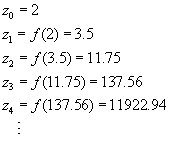We will refer to a complex number z0 as a prisoner if its orbit under f is bounded, and an escapee if the orbit is unbounded -- that is, terms in the orbit get arbitrarily far away from 0.  The set of all prisoners for a given function f is called its prisoner set, and the set of all escapees is called the escape set.

In the above examples, we used complex numbers z0 for which the imaginary part is 0, just to simplify the calculations.  But the analogous calculations can be done for any complex value of z0 , using the multiplication and addition of complex numbers as discussed in Vignette 10.  For example, for the function, the orbit of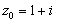isIn this example, we see thatis in the prisoner set for, because its orbit is bounded.

Julia Sets

Julia was interested in the properties, for various functions, of the prisoner set and the escape set -- and also what is now called the Julia set for the function.  The Julia set is defined to be the boundary between the prisoner set and the escape set.  The Julia set for consists of the points   In order to determine these sets experimentally, it is necessary to compute the orbits of a great many starting points z0 , since we must know the orbit in order to determine whether the starting point is a prisoner or an escapee.  And if we had to do the calculation of orbits such as the last one above purely by hand -- for several thousand starting values -- we would quickly lose interest in the project!

That's where mathematical theory comes into play.  Even without actually doing all of the tedious calculations, a number of things can be proved about prisoner sets, escape sets and Julia sets.  But rather than looking at the theory, we will instead consider the fact that, starting in the 1980's, the availability of computers led people back to the idea of a visual exploration of Julia sets -- with incredibly beautiful results.  After all, a computer could be programmed to do the calculation of an orbit of a starting value of  z0 -- and would not even object if asked to do a similar calculation for thousands or even millions of such similar values!

For simplicity, let's consider only functions of the form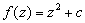, where c is some fixed complex number.  It can be proven that if the orbit of a starting value z0 ever leaves the circle of radius 2 centered at the origin, then the rest of the terms in the orbit get successively farther from 0, and in fact the orbit is unbounded.  Such a starting value  z0 would therefore be an element of the escape set for f .  Armed with this simple mathematical theorem and a good bit of computer power, we are ready to visualize the prisoner set and escape set for a given function of the form.  The Julia set can then be pictured as the boundary between the two sets.

Visualizing Julia Sets

To draw a picture of a Julia set, we imagine the computer screen as being a "window" showing a portion of the complex number plane.  (Usually this portion is only a few units in each direction, because that's where the interesting features are.)  We decide on a function to use -- by fixing the value of c in the formula.  For each of the points on the screen that the computer is capable of using -- these are called pixels -- we convert that pixel to the corresponding complex number, and then use that complex number as the starting value z0 .  The computer then computes terms in the orbit of that  z0 until one of the terms is outside the circle of radius 2 (in which case z0 is known to be an escapee), or until some previously-set number of terms has been calculated -- say 200 terms. (In the latter case, the orbit has not gone outside the circle of radius 2 in the first 200 terms, so it is a pretty safe bet -- although not absolutely certain -- that  z0 is a prisoner.)  The pixel corresponding to z0 is then colored on the computer screen according to some chosen coloring scheme.  A commonly used coloring scheme is to color prisoner points black, while assigning escapees a color to indicate how soon the orbit left the circle of radius 2.

The images below show portions of the prisoner and escape sets for the various functions indicated.  Coloring was done using the scheme described above, so in each case, the Julia set is the boundary between the black region and the colored regions.  In some cases, the extreme portions of the escape set may also be colored black, to draw attention to the more interesting regions of the escape set.  In images where you do not see a definite black region, it is either because the image shows only a portion of the escape set, or because the portions of the prisoner set are so small that they are in between pixels that the computer has used, and therefore are overlooked.  In each case, you can click on the thumbnail image to see an enlarged version.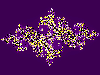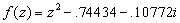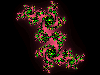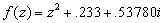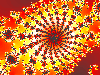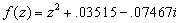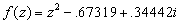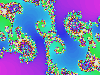In looking at the larger versions of some of these images, you have undoubtedly noticed a type of self-similarity -- that is, the structure and shape of the image is repeated throughout smaller portions of the image.  This self-similarity is characteristic of Julia sets, making them examples of fractals.  From a mathematical point of view, however, the real importance of Julia sets lies in the fact that the Julia set for a function f is the set of points on which  f  acts chaotically --   small distances between points in the Julia set are magnified under iteration of f , so that even a tiny error in locating a given point can be magnified to the extent that long-range predictions about the resulting value are hopeless.  (This is the same phenomenon discussed in Vignette 13 on population models.)

Further Exploration

• A Gallery of Julia Sets
• Fractint Web Pages (Public domain software to produce many types of fractal images, including Julia sets)
• Ivars Peterson, The Mathematical Tourist: Snapshots of Modern Mathematics.  In JCU Library, call number  QA93 .P475
• Robert L. Devaney, A First Course in Chaotic Dynamical Systems.  In JCU Library, call number QA614.8.D49
• Heinz-Otto Peitgen et al., Chaos and Fractals: New Frontiers of Science.  In JCU Library, call number QA614.86 .P43